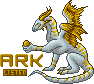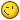# Belisar

Members

3,108

• #### Last visited

• Rank
To boldly float where no one has floated before >:3• Birthday June 22

## Signature

PLEASE NOTE: AM ON KIND OF A BREAK - ONLY ONLINE FROM TIME TO TIME - WILL STILL FULLFILL MY IOUs - NO WORRIES :)~*~ WISH and BREEDING LISTs ~*~All your BALLOON are belong to ... ME!=> Always searching for 2nd gen Balloons from Spriter Alts/Prize/HM/female Holly/Black Sweetling/April Fools Dilemma Balloon or 2nd gen Prize from x Balloon - Please PM me if you can breed such, I do my best to find you something you like in return *-*

## Profile Information

• Gender
Female
• Location
Behind a tree
• Interests
~~~~~~~~~~~ <3 WISHLIST <3 ~~~~~~~~~~~
-> IF YOU WANT TO MAKE ME REALLY HAPPY AND HELP WITH MY LOON HOARDING ISSUES:

* 2nd gen Balloon from x Spriter Alt

* 2nd gen from male + female Alt Pumpkin

* 2nd gen Balloon from "Honorable Mention" CB Hybrid/Bred-only Alt/female Holly

* 2nd gen Holly from female Holly x Balloon

* 2nd gen Prize from Prize x Balloon

* 2nd gen Balloon from x Prize dragon

* 2nd gen Balloon from April Fool's day "Cave" Balloon

* 2nd gen Balloon from Upside Down April Fool Mint

* 2nd gen Balloon from Black Sweetling

* CB Balloon

~~~~~~~~~~~~~ CAN OFFER ~~~~~~~~~~~~
*~ CB breedings of all sorts ~*
*~ catch and hatch on your command ;) ~*

((((((((((((((((((((((((((((((((((((^_^))))))))))))))))))))))))))))))))))))))

~~~~ HAVE HOLIDAY BREEDINGS ~~~~
* Breeding 2nd gens from my original CB Holiday Dragons
[with mate of your choice - can provide everything but CB Prize or HM - during their breeding time]

~~~~~~~~~~~~~ WANT ~~~~~~~~~~~~~
-> CB Balloon Eggs/Hatchlings
-> Holiday swap [probably from x Balloon]

((((((((((((((((((((((((((((((((((((*_*))))))))))))))))))))))))))))))))))))))

~~NEED LINEAGE MATES~~

~~~~~~ CAN OFFER ~~~~~~
*~ CB breedings of all sorts ~*
*~ catch and hatch on your command ;) ~*

((((((((((((((((((((((((((((((((((((^_~))))))))))))))))))))))))))))))))))))))

~~~~ WE OWE EACHOTHER: ~~~~
* snakehands [Jan19]: my 2nd gen Silver from Starsinger for your 2nd gen Balloon from female Upsidedown Mint

~~~~ I OWE YOU: ~~~~
* Fiona: a free ticket to help with anything she needs

* hellhunt [April19]: 110 CB+EG Pygmy Hatchies for 3 ND Hatch [81/110 done]

* Wiz [Mar19]: 3rd gen Stair Tinsel from Gold Tin x Loon

((((((((((((((((((((((((((((((((((((0_0))))))))))))))))))))))))))))))))))))))

~~~~ YOU OWE ME ;) : ~~~~
* Lorimmel : a 2nd gen Balloon from your Prize for my Neglected Hatchling - I paied my share; as it's been 6 years and several reminders guess IOU failed :(

* WaterDroplet/Vibrance [May 2017]: my CB Silver (1/1) for 1 of each CB Xenowyrm and "couple of" CB Balloon Hatch: 1/1 Pyro, 1/1 Thalassa, 1/1 Astrapi, 0/1 Gaia, 1/1 Mageia, 0/1 Chronos, Balloon hatch 4/10 -> picked up with IOU again :)

~~~~~~MORE BREEDING INFOS/LISTS in the LINK in my SIG!~~~~~~